# LOG#127. Basic Neutrinology(XII).When neutrinos pass through matter or they propagate in a medium (not in the vacuum), a subtle and potentially important effect occurs. This is called the MSW effect (Mikheyev-Smirnov-Wolfenstein effect). It is pretty similar to a refraction of light in a medium, but now it happens that the particle (wave) propagating are not electromagnetic waves (photons) but neutrinos! In fact, the MSW effect consists in two different effects:

1st. A “resonance” enhancement of the neutrino oscillation pattern.

In the presence of matter, the neutrino experiences scattering and absorption. This last phenomenon is always negligible (or almost in most cases). At very low energies, coherent elastic forward scattering is the most important process. Similarly to optics, the net effect is the appearance of a phase difference, a refractive index or, equivalently, a neutrino effective mass.

The neutrino effective mass can cause an important change in the neutrino oscillation pattern, depending on the densities and composition of the medium. It also depends on the nature of the neutrino (its energy, its type and its oscillation length). In the neutrino case, the medium is “flavor-dispersive”: the matter is usually non-symmetric with respect to the lepton numbers! Then, the effective neutrino mass is different for the different weak eigenstates!

I will try to explain it as simple as possible here. For instance, take the solar electron plasma. The electrons in the solar medium have charged current interactions withbut not with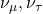. Thus, the resulting interaction energy is given by a interaction hamiltonian

(1)where the numerical prefactor is conventional,is the Fermi constant and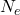is the electron density. The corresponding neutral current interactions are identical fo al the neutrino species and, therefore, we have no net effect on their propagation. Hypothetical sterile neutrinos would have no interaction at all either. The effective global hamiltonian in flacor space is now the sum of two terms, the vacuum hamiltonian and the interaction part. We can write them together

(2)The consequence of this new effective hamiltonian is that the oscillation probabilities of the neutrino in matter can be largely increased due to a resonance with matter. In matter, for the simplest case with 2 flavors and 2 dimensions, we can define an effective oscillation/mixing angle as

(3)The presence of the term proportional to the electron density can produce “a resonance” nullifying the denominator. there is a critical densitysuch as

(4)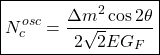for which the matter mixing angle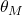becomes maximal and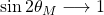, irrespectively of the value of the mixing angle in vacuum. The probability thatoscillates or mixes into aweak eigenstate after traveling a distance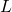in this medium is give by the vacuum oscillation formula modified as follows:

1st.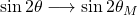2nd. The kinematical factor differs by the replacement of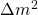with. Hence, it follows that, at the critical density, we have the oscillation probability in matter (2 flavor and 2 dimensions):

(5)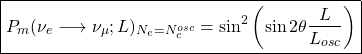This equation tells us that we can get a full conversion of electron neutrino weak eigenstates into muon weak eigenstates, provided that the length and energy of the neutrino satisfy the condition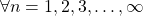There is a second interesting limit that is mentioned often. This limit happens whenever the electron densityis so large such that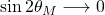, or equivalently,. In this (dense matter) limit, there are NO oscillation in matter (they are “density suppresed”) because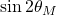vanishes and we haveTherefore, the lesson here is that a big density can spoil the phenomenon of neutrino oscillations!

In summary, we have learned here that:

1st. There are neutrino oscillations “triggered” by matter. Matter can enhance or enlarge neutrino mixing by “resonance”.

2nd. A high enough matter density can spoil the neutrino mixing (the complementary effect to the previous one).

The MSW effect is particularly important in the field of geoneutrinos and when the neutrinos pass through the Earth core or mantle, as much as it also matters inside the stars or in collapsing stars that will become into supernovae. The flavor of neutrino states follows changes in the matter density!

See you in my next neutrinological post!

claimtoken-521a433ae2380

This site uses Akismet to reduce spam. Learn how your comment data is processed.